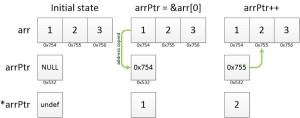# Pointer Arithmetic

Do you want to be a Master in C? then you should have to know about pointer arithmetic.

Referencing a Pointer:

• Referencing the pointer means storing the address of particular object into the pointer variable.

For ex:

int no = 10;
int *ptr = & no;

• In this example we store the address of no variable in a pointer variable ptr. & operator is used for retrieving the address and it is called as “address of operator “.

• This “&” operator is not used for an array and a function names because name of an array and function itself is the address.

Consider the example of a function pointer:

int Fun(int , char);
int (*fptr)(int , char);
fptr = Fun; // Here there is no need to use the & operator.

• Important point is that we can’t store a constant value directly inside the pointer variable like

int *ptr = 1859; // It gives error

Dereferencing a Pointer :
• Dereferencing the pointer means, accessing the contents at which our pointer is pointing, means access the contents at an address which is stored in our pointer variable.
For ex:
int no = 21;
int * ptr = &no;
printf(“%d”,*ptr); // 21

• In this example, we are able to access the contents whose address is stored in pointer ptr.
• Number of a bytes derefer by a pointer is depend on the type of a pointer but it is does not depend on the pointed contents.
• Means if our pointer is of character pointer, it can access 1 bytea and if our pointer is of integer pointer, it can access only 4 bytes.

Arithmetic operations on pointers:
We can add an integer constant with the pointer variable.

For ex:
int no = 51;
int *p = &no;

suppose an address of no variable is 100, means pointer p stores address 100.

p = p +2;
Result = pointer value + integral constant * size of pointer type.

Which evaluates as
p = 100 + (2 * 4);
p = 100 + 8;
p = 108;

Now our pointer is pointing at address 108.

Addition of a pointer and an integer is commutative means
(p + 2) is same as (2 + p)

We can not add tow pointers means
int no1 = 21, no2 = 51;
int *p = &no1;
int *q = &no2;
p + q; //It gives errorIncrement operation on pointer:
• C provides “++” as a increment operator.
• We can apply this “++” operator on a pointer variable also.
• Effect of this “++”operator is same as addition of pointer with 1.

For ex:
double d = 3.15;
double *p = &d;
p++;
p++ is evaluates as
p = p + size of pointer type

suppose in our example p is pointing at address 200 then after incremente the operation it points at 208. It can be simplified as
p = 200 + 8;
p = 208;
Subtraction operation :
We can subtract constant integer value from the pointer by applying same rule as a addition operation.

For ex:
float no = 7.8;
float *p = &no;

suppose an address of variable no is 8000, means pointer p stores address 8000.

p = p – 2;
Result = pointer value + integral constant * size of pointer type.

This evaluates as
p = 8000 – (2 * 4);
p = 8000 – 8;
p = 7992;

Now our pointer is pointing to an address 7992.
Subtraction of the pointer and an integer is not commutative means
(p + 2) is not same as (2 + p). (2 + p) is illegal operation.

• Two pointers can also be subtracted but this is only meaningful when both the pointers are pointing to the elements of same array.
• Then the result of it will be difference subscripts of two array elements.

For more reading about technology news in singapore and seo to online marketing do view more about other pages.#### Sourabh Bhunje

Sourabh Bhunje, B.E. IT from Pune University. Currently Working at Techliebe. Professional Skills: Programming - Software & Mobile, Web & Graphic Design, Localization, Content Writing, Sub-Titling etc. http://techliebe.com/about-us